# OUTER ELECTRONS VALENCE ELECTRONS The electrons in the

• Slides: 34OUTER ELECTRONSVALENCE ELECTRONS The electrons in the outermost energy levels of the atom are involved in chemical reactions They are the ones that bond with other atoms These outermost e- are called valence electrons Valence electrons: an electron in the outermost energy level of an atom.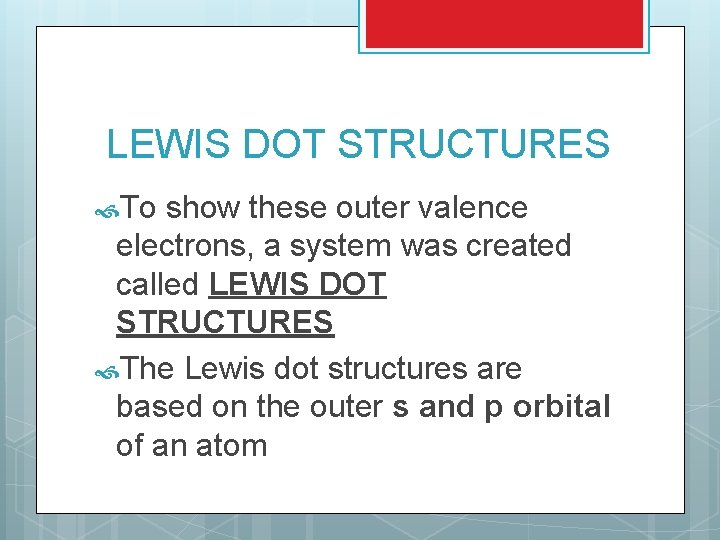LEWIS DOT STRUCTURES To show these outer valence electrons, a system was created called LEWIS DOT STRUCTURES The Lewis dot structures are based on the outer s and p orbital of an atom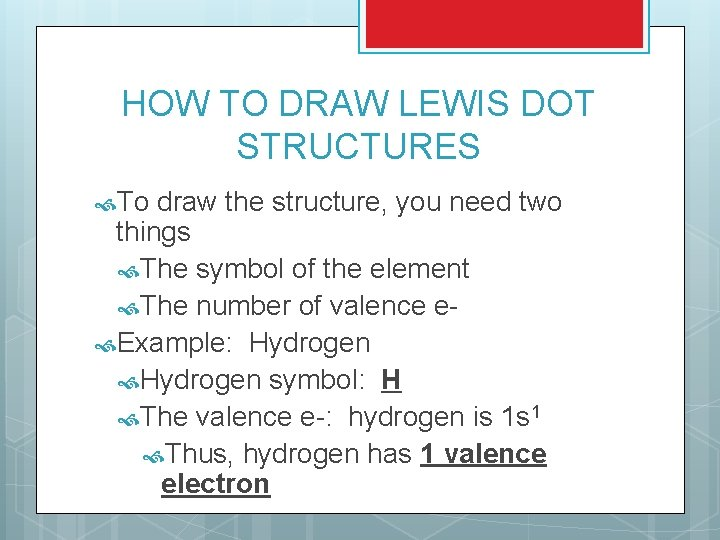HOW TO DRAW LEWIS DOT STRUCTURES To draw the structure, you need two things The symbol of the element The number of valence e Example: Hydrogen symbol: H The valence e-: hydrogen is 1 s 1 Thus, hydrogen has 1 valence electron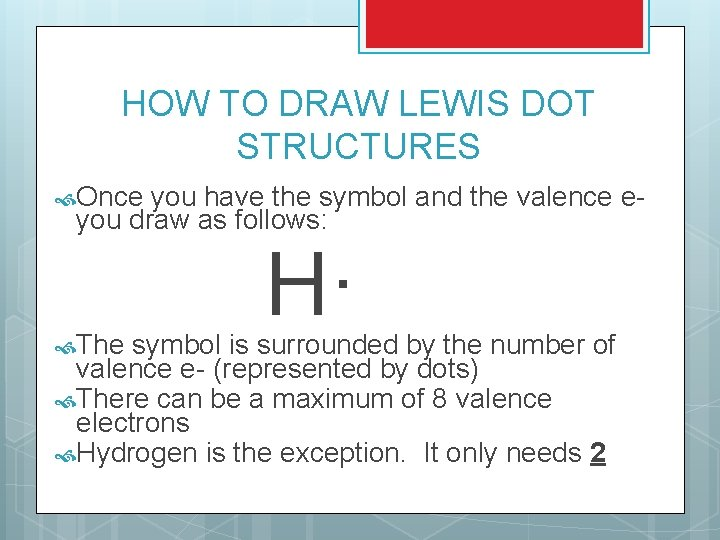HOW TO DRAW LEWIS DOT STRUCTURES Once you have the symbol and the valence eyou draw as follows: The H· symbol is surrounded by the number of valence e- (represented by dots) There can be a maximum of 8 valence electrons Hydrogen is the exception. It only needs 2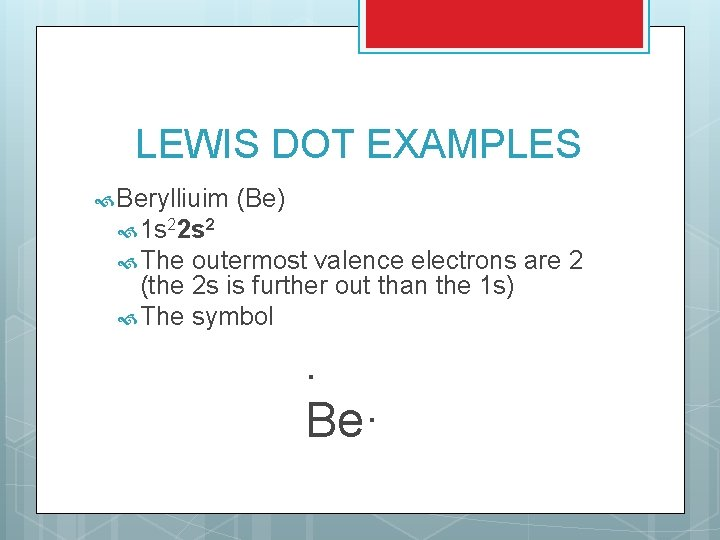LEWIS DOT EXAMPLES Berylliuim (Be) 1 s 22 s 2 The outermost valence electrons are 2 (the 2 s is further out than the 1 s) The symbol . Be·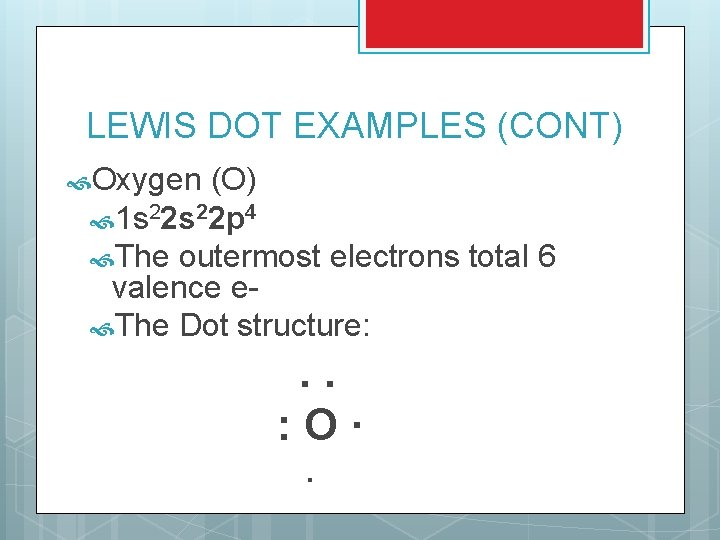LEWIS DOT EXAMPLES (CONT) Oxygen (O) 1 s 22 p 4 The outermost electrons total 6 valence e The Dot structure: . . : O· ·TRY THESE 1. 2. 3. 4. 5. Boron (B) Carbon (C) Nitrogen (N) Fluorine (F) Neon (Ne)SHORT CUT Examples on using the periodic tableHYDROGEN (THE SPECIAL CASE) In order for hydrogen to be stable, it needs to have the s orbital filled (2 e-) This can be done by sharing electrons Example: H· + H· H : H When the electrons are shared, you symbolize with a (-) H : H H - H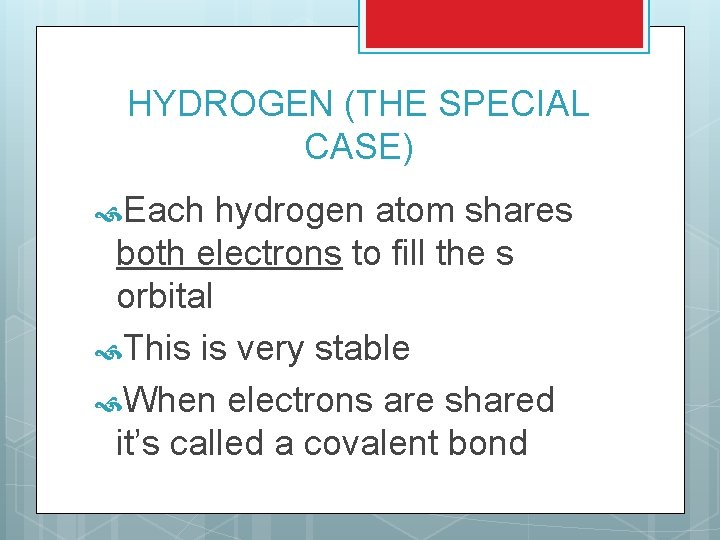HYDROGEN (THE SPECIAL CASE) Each hydrogen atom shares both electrons to fill the s orbital This is very stable When electrons are shared it’s called a covalent bond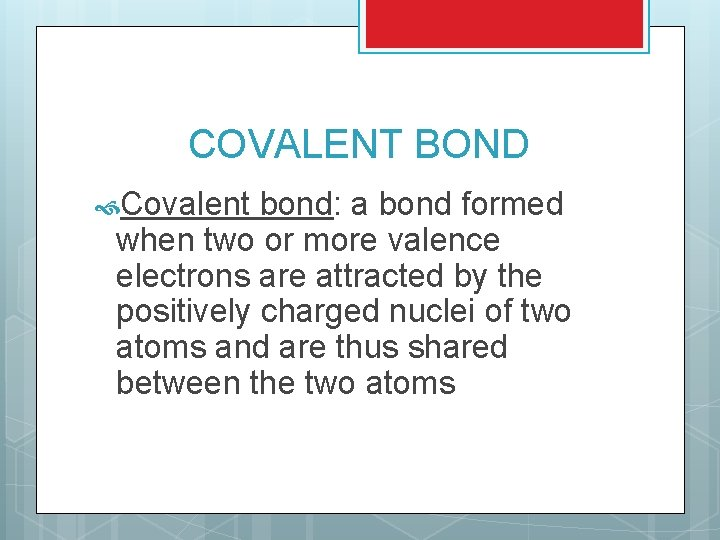COVALENT BOND Covalent bond: a bond formed when two or more valence electrons are attracted by the positively charged nuclei of two atoms and are thus shared between the two atoms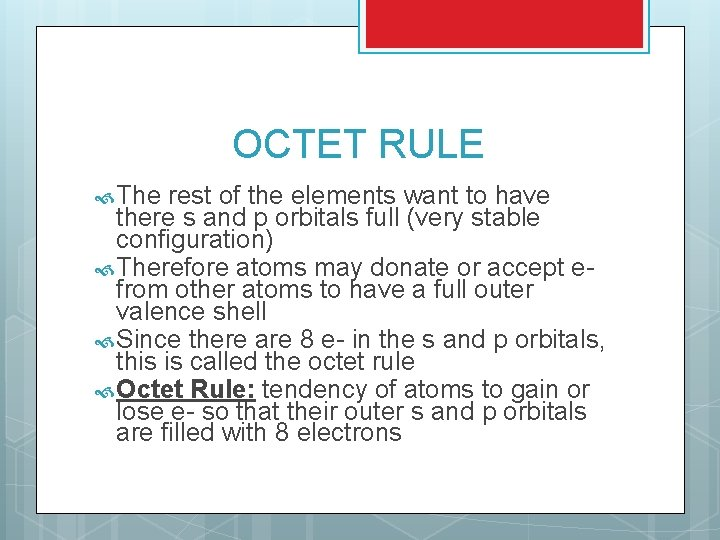OCTET RULE The rest of the elements want to have there s and p orbitals full (very stable configuration) Therefore atoms may donate or accept efrom other atoms to have a full outer valence shell Since there are 8 e- in the s and p orbitals, this is called the octet rule Octet Rule: tendency of atoms to gain or lose e- so that their outer s and p orbitals are filled with 8 electronsConsider BONDING BETWEEN ELEMENTS the chlorine (Cl) atom ·· : Cl· ·· When chlorine binds with another chlorine, you get ·· ·· ·· ·· : Cl: : Cl – Cl: ·· ·· ·· ·· When a pair of electrons are shared they form a single bond (look for binding sites) The other three pairs around the chlorine atom are called the unshared pair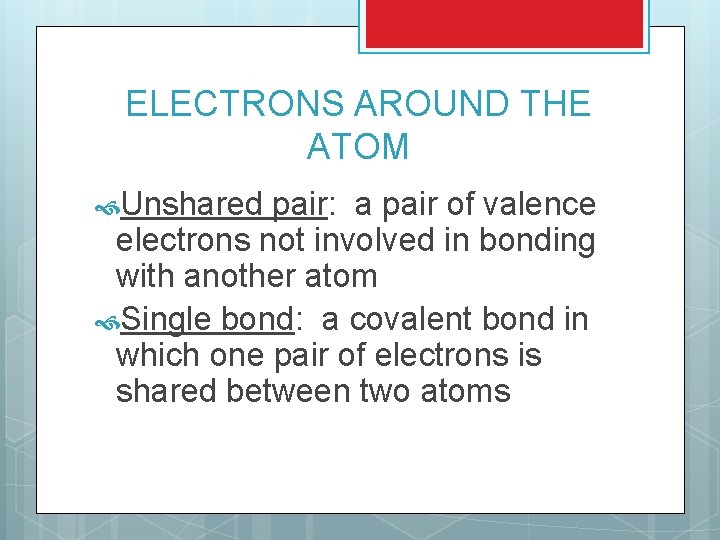ELECTRONS AROUND THE ATOM Unshared pair: a pair of valence electrons not involved in bonding with another atom Single bond: a covalent bond in which one pair of electrons is shared between two atomsTRY THESE 1. 2. 3. 4. Draw the Lewis structures between the following elements H+I I+I H + Cl H+FRULES FOR DRAWING LEWIS STRUCTURES So far we’ve done some simple Lewis dot structures, but how do you draw more complex structures such as CH 3 I Step 1: Find the central atom and determine the total valence electrons in the compound. Draw each Lewis structure separately Add up the total valence e- of each elementTOTAL NUMBER OF VALENCE ELECTRONS CH 3 I Carbon (C) has 4 valence e- = 4 3 Hydrogen (H) with 1 e= 3 Iodine (I) has 7 valence e= 7 14 electrons NOTE: Carbon has the most number of open valence e- (the central atom).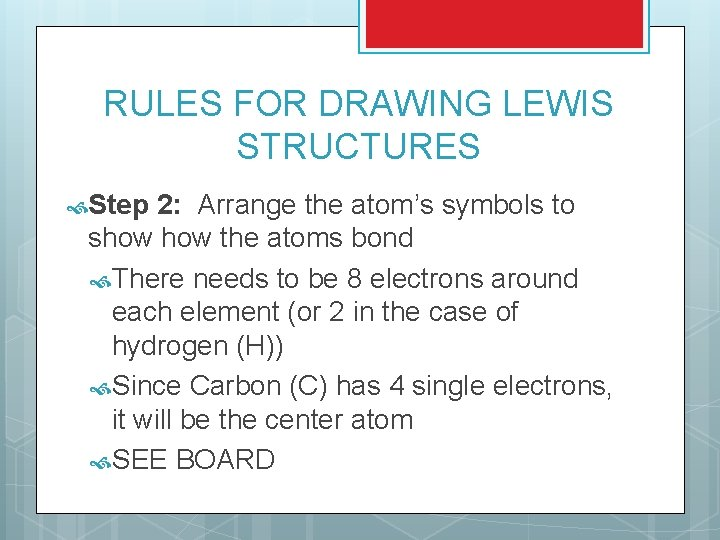RULES FOR DRAWING LEWIS STRUCTURES Step 2: Arrange the atom’s symbols to show the atoms bond There needs to be 8 electrons around each element (or 2 in the case of hydrogen (H)) Since Carbon (C) has 4 single electrons, it will be the center atom SEE BOARD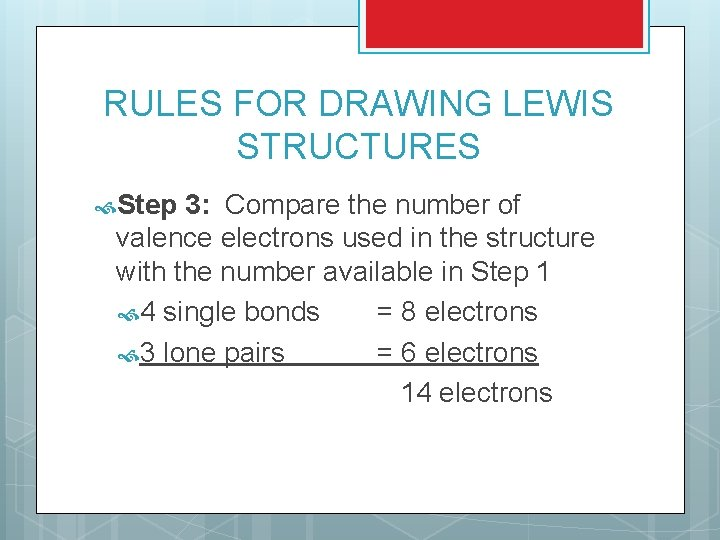RULES FOR DRAWING LEWIS STRUCTURES Step 3: Compare the number of valence electrons used in the structure with the number available in Step 1 4 single bonds = 8 electrons 3 lone pairs = 6 electrons 14 electronsRULES FOR DRAWING LEWIS STRUCTURES Step 4: Change each pair of dots that represent a shared pair of electrons to a single dash Step 5: Be sure that all atoms, with the exception of hydrogen (H), follow the octet rule.TRY THESE 1. 2. 3. 4. CH 3 I CH 2 Cl 2 Br. F C 2 H 6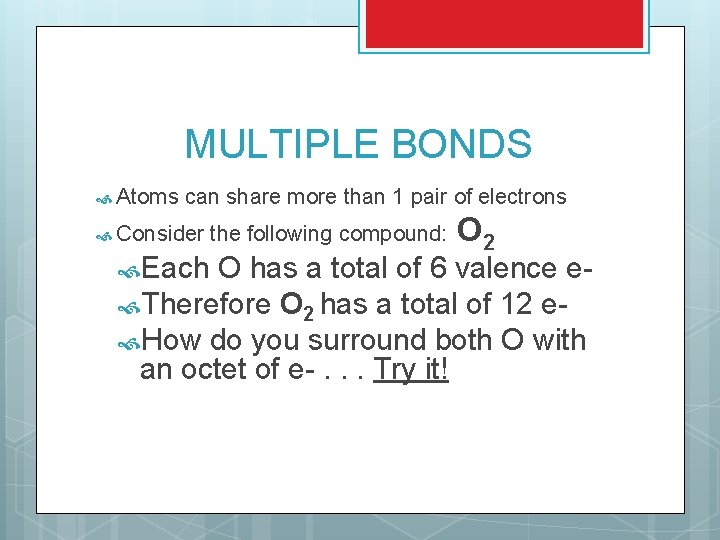MULTIPLE BONDS Atoms can share more than 1 pair of electrons Consider Each the following compound: O 2 O has a total of 6 valence e Therefore O 2 has a total of 12 e How do you surround both O with an octet of e-. . . Try it!MULTIPLE BONDS To get each atom to have an octet of electrons, the O must share 2 pairs of electrons When compounds share 2 pairs of electrons, it is called a double bond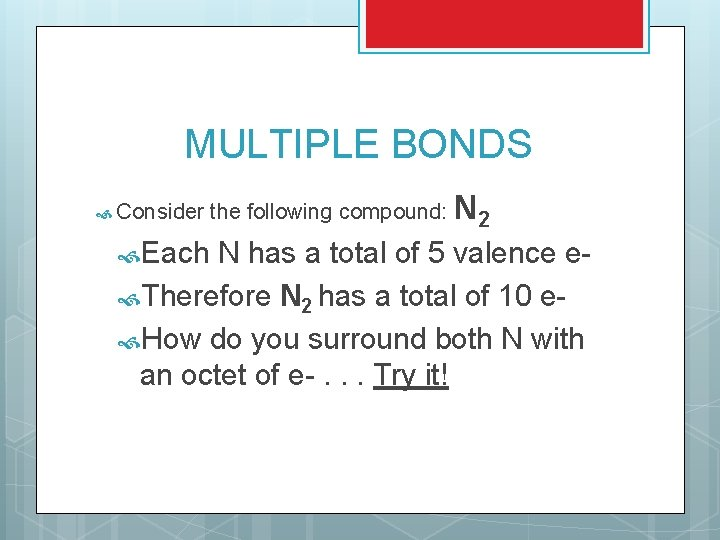MULTIPLE BONDS Consider Each the following compound: N 2 N has a total of 5 valence e Therefore N 2 has a total of 10 e How do you surround both N with an octet of e-. . . Try it!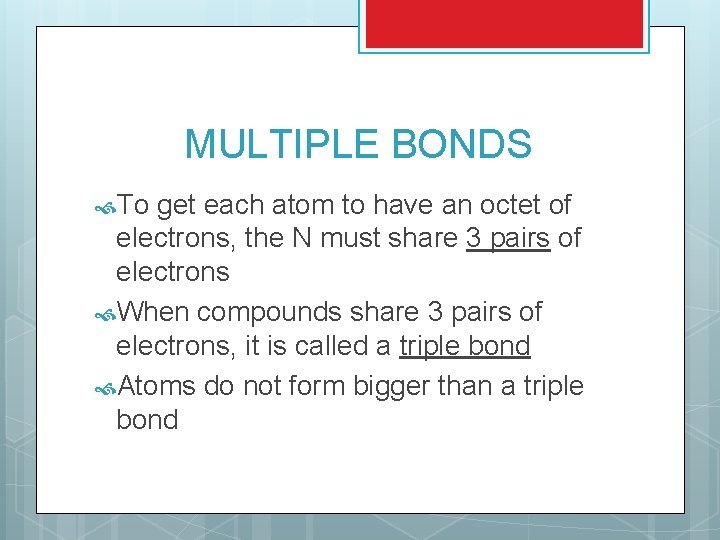MULTIPLE BONDS To get each atom to have an octet of electrons, the N must share 3 pairs of electrons When compounds share 3 pairs of electrons, it is called a triple bond Atoms do not form bigger than a triple bondTRY THESE 1. 2. 3. CO 2 C 2 H 4 HCN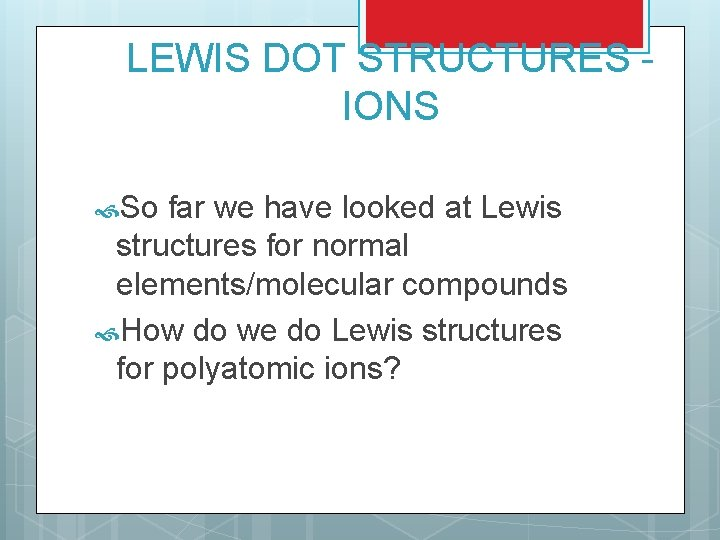LEWIS DOT STRUCTURES IONS So far we have looked at Lewis structures for normal elements/molecular compounds How do we do Lewis structures for polyatomic ions?ANSWER Whenever you have a negative “-” charge, you add that many electrons Whenever you have a positive “+” charge, you subtract that many electrons For example SO 4 -2 Normally: 1 S = 6 e 4 O = 4 x 6 e- = 24 e Total = 30 e-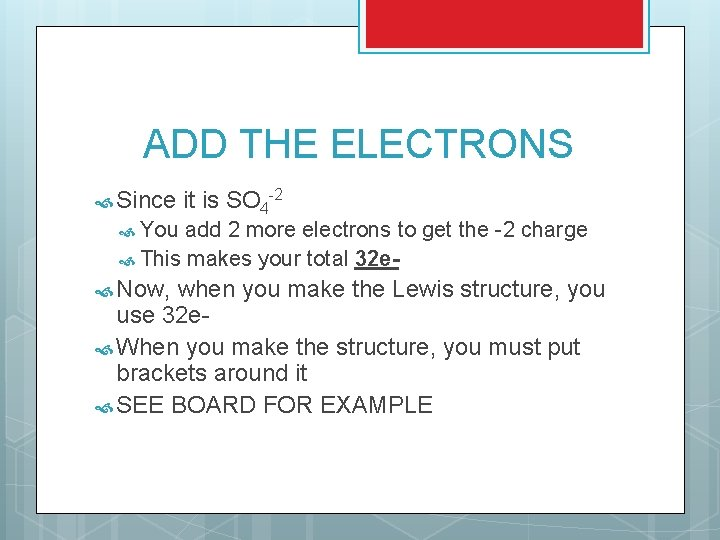ADD THE ELECTRONS Since it is SO 4 -2 You add 2 more electrons to get the -2 charge This makes your total 32 e Now, when you make the Lewis structure, you use 32 e When you make the structure, you must put brackets around it SEE BOARD FOR EXAMPLETRY THESE 2. Cl. O 4 NH 4+ ADDITIONAL PRACTICE 1.RESONANCE STRUCTURES Sometimes the double bond does not exist between just 2 elements. They sometimes form Resonance structures A possible Lewis structure of a molecule for which more than one Lewis structure can be written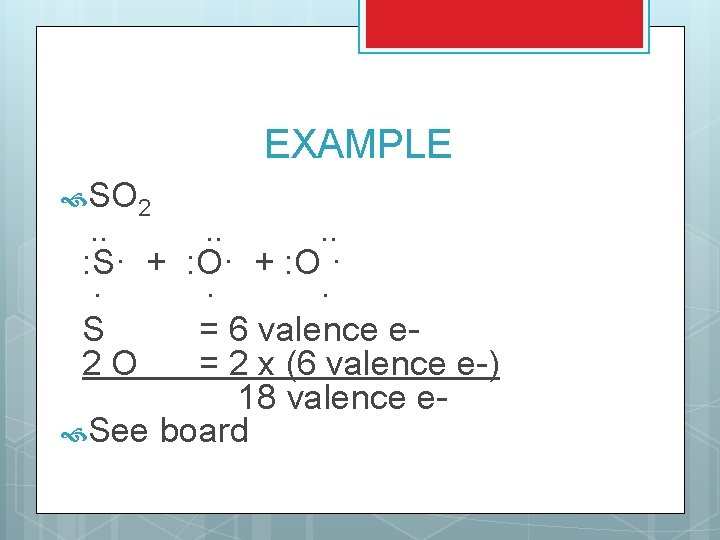EXAMPLE SO 2 . . . : S· + : O · · S = 6 valence e 2 O = 2 x (6 valence e-) 18 valence e See board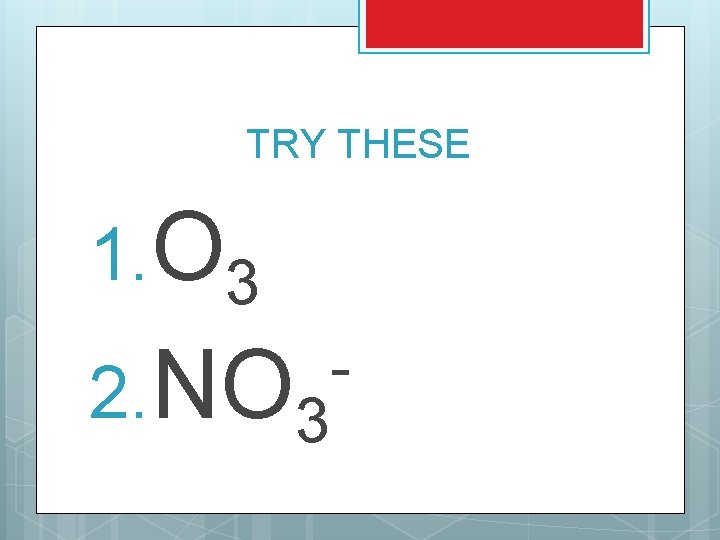TRY THESE 1. O 3 2. NO 3 -